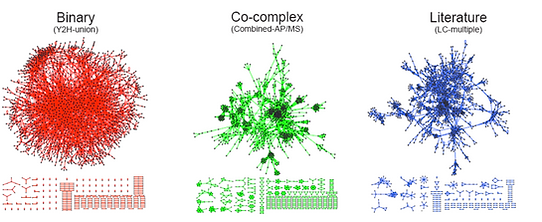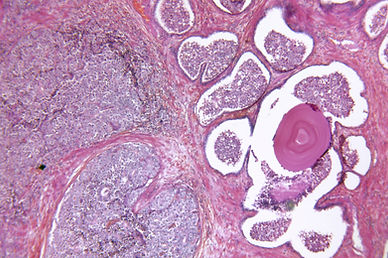top of page## Assignments on Machine Learning and Data Mining## Neural Processes for Ejection Fraction Estimation from Echocardiograms

Neural Processes (NP) are a family of machine learning algorithms that combine meta-learning and stochastic processes to introduce a new paradigm in probabilistic machine learning. NPs are computationally expensive, and using them for video data requires innovations to how the videos are encoded. In this project, we enable meta-learning for ejection fraction estimation from noisy medical ultrasound videos of the heart by introducing intermediate latent variables to encode video content.## Graph Generation Classification Network

I explored Graph Autoencoders (GAE) to generate a graph structure from input ECG signals and then classify the graph to assess cardiac function. More specifically, the Graph Generation Classification Network combines the graph generation and classification processes together. The generation step involves a Variational Graph Autoencoder to create graphs prior to the classification step using Graph Neural Networks. Both the classifier and generator are trained together using a joint objective function. With this approach, graphs can be created for medical datasets without reliance on metadata such as patients' age and gender.## Assignments on Probabilistic Programming

My solutions for a set of assignments covering fundamental topics of probabilistic programming as taught by Dr. Frank Wood.## Assignment on Introduction to Graph Neural Networks

I designed an assignment including theoretical questions and a hands-on project providing an introduction to Graph Neural Networks. The project transforms MNIST digit images into grid graphs prior to performing graph classification to classify each image into a digit.## Binary Classification of ECG Signals using K-Nearest Neighbor Graphs

In this project, 15-node K-Nearest Neighbour (KNN) graphs are created with each node corresponding to one channel of ECG data. Graph Neural Networks (GNNs) are used to generate embeddings from these graphs, which are then used for classification. The purpose of this experiment is to explore alternatives to using medical metadata (such as patients' age or gender) in creating graphs prior to medical diagnosis tasks.## Assignments on Advanced Machine Learning

My solutions for a set of assignments covering advanced machine learning topics as taught by Dr. Mark Schmidt.## Graph Convolutional Neural Networks for Edge Prediction of Yeast Protein Interactions

A PyTorch re-implementation of Stanford's tutorial on GCN for edge prediction## Prostate Cancer Classifier Using Graph Neural Networks

This project aims to predict whether prostate biopsy cores are cancerous or healthy using Graph Neural Networks. Data is not originally in graph form, and the graphs are created using the input FFT data. Time-domain RF data is then used as node features.## Node Skip: A Node Dropout Algorithm to Increase GNNs Generalizability

In this project, I explored a novel node dropout algorithm where nodes are randomly dropped, and edges are added to the graph to avoid the introduction of disconnected components after node dropout. Additionally, the added edges allow higher order neighbor communication using a lower number of GNN layers.bottom of page